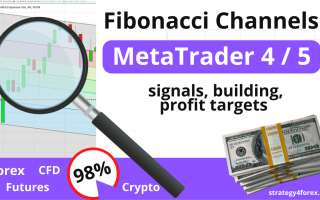# Fibonacci Channels — signals, building, profit targets

Today we look at the tool Metatrader 4, by which we can predict the price of a stopping point on a chart or a trend reversal — Channels Fibonacci, for which we define the Fibonacci Channels are needed, how they build on the currency pair and set up a trading platform Metatrader 4.

And also Fibonacci channels can be used as target levels, similar to the usual equidistant channels, which we reviewed earlier HERE ➜

Decided to publish this tutorial forex, because occasionally get questions from readers on their sites about the use of parallel channels and channels Fibonacci forex as well.

• Fibonacci Channel is a system of parallel trend lines: the main channel + equidistant parallel lines in the proportions of the Fibonacci series: 61.8%, 161.8%, 200%, 261.8%, etc.

Fibonacci Channel is necessary to build a minimum of 2 meters and the extremes to hold a parallel line through it, eventually you will have a projection of the trend lines with the Fibonacci ratio.

## Drawing lines of the Fibonacci Channel:

If you build the upward channel Fibonacci, then build it in two successive maxima and conduct a parallel line to a minimum, the result will be constructed projection of Fibonacci trend lines: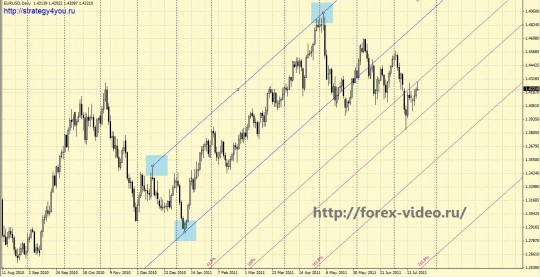If you are building a downlink Fibonacci, then build it through two consecutive minimum and holding at least a parallel line on the 1 st to the maximum, the result will be constructed projection of Fibonacci trend lines: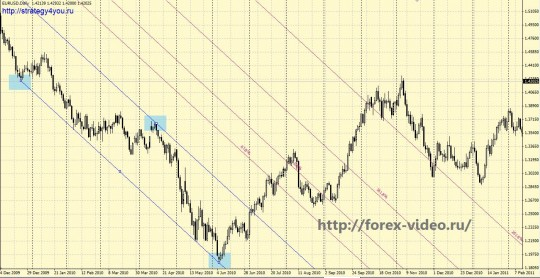Constructed parallel to the channel line Fibonacci 61.8%, 161.8%, 200%, 261.8%, etc. will be a further price movement support and resistance lines on which the price may bounce or breaking the Fibonacci level, will give the signal for the transaction, in the presence of other signals on the chart for the chosen currency pair.

You can also combine the channels and the Fibonacci sequence as constructed in the same direction or in opposite directions, we end up with the important points of intersection of Fibonacci projections or significant accumulations of Fibonacci trendlines.

## Here are a couple more examples of using fibo channels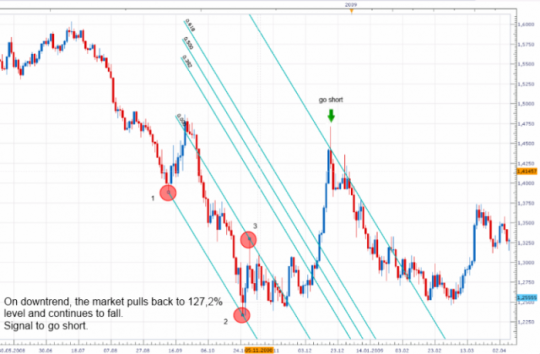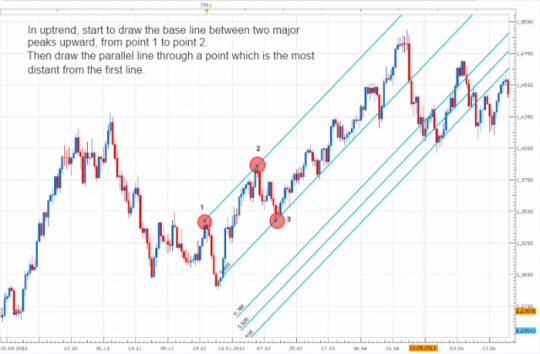### Video lesson «Building Fibonacci Channels»:

• Recommended to watch with English subtitles!

As for me, I almost do not use this tool in your MT4 forex trading, as I think that these channels do not work so well as themselves, and Fibonacci extension levels, as well as equidistant channels forex.

Original